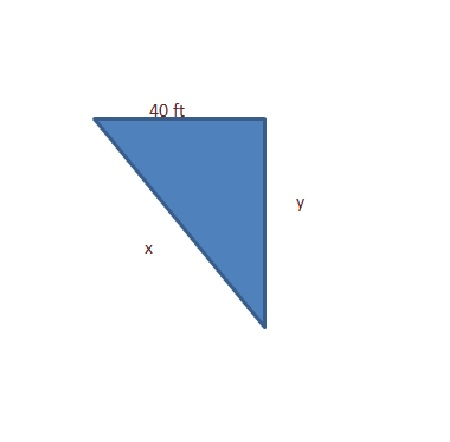# A police car is located 40 feet to the side of a straight road. A red car is driving along the...

## Question:

A police car is located 40 feet to the side of a straight road.

A red car is driving along the road in the direction of he police car and is 90 feet up the road from the location of the police car. Tthe police radar reads that the distance between the police car and the red car is decreasing at a rate of 100 feet per second. How fast is the red car actually traveling along the road?

## Finding the Speed of the Car:

We need to find the speed of the red car actually traveling along the road. So we need to find the {eq}\frac{dy}{dt} {/eq}. We know the Pythagoras theorem,

{eq}Hypotenuse^2 = opposite^2 + adjacent^2 {/eq}

Become a Study.com member to unlock this answer!By Pythagoras theorem,

{eq}x^2=y^2+(40)^2 \\x^2=y^2+1600 \quad \cdots Equation \ (1) {/eq}

Lets differentiate with respect to {eq}t {/eq} :

...The Pythagorean Theorem: Practice and Application

from

Chapter 14 / Lesson 6
68K

Learn what the Pythagorean theorem is. Discover how to prove and use the Pythagorean theorem with examples, and identify how this theorem is used in real life.